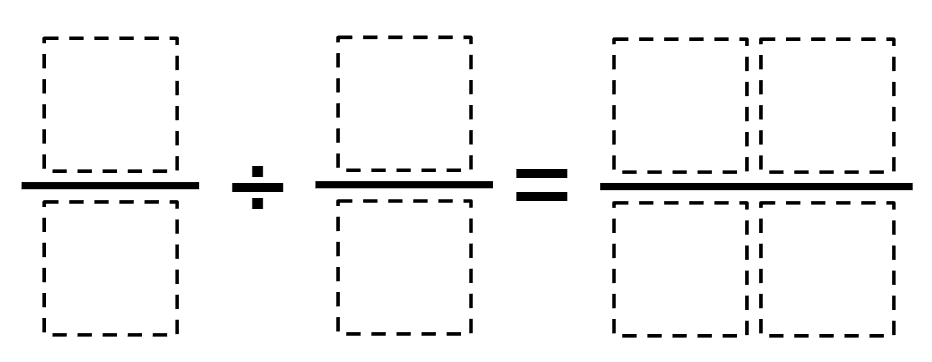# Dividing Fractions

Directions: Using the digits 0 to 9 at most one time each, place a digit in each box to make a true fraction division sentence.### Hint

Think about the process you think through to divide fractions. How can you move your fractions around so that you can determine the numerator and denominator of the answer?

There are many possible answers including:
3/5 ÷ 8/9 = 27/40
7/5 ÷ 8/3 = 21/40
8/1 ÷ 6/3 = 45/90

Source: Shanelia Rhome-Shannon and Aiden (Student)

## Percent Of A Quantity 3

Directions: Using the digits 0 to 9 at most one time each, place a digit …

1.Diana Khutsaeva

2/6: 5/4=8/30

2.Okay this maybe a stupid question, but isn’t open middle supposed to have a correct answer? Like 1 correct answer?

•no……there are sometimes many even in some cases infinite

•I just now listened to Robert’s seminar and he specifically said one answer. He said open ended has many answers. So yes. You are corrrct.

3.i think it’s 3/5 ÷ 8/9 = 27/40

4.3/6 divided by 9/7 = 21/54

5.3/4 divided by 5/6 = 18/20

6.You could make it have one answer by saying it has to be the greatest or least quotient possible or something like that.